# Direction of E field and propagation of wave in a conductor

• I
iVenky
We know that skin depth in a conductor is found using the following expression,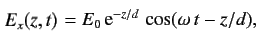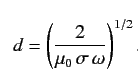(Credits: http://farside.ph.utexas.edu/teaching/315/Waves/node65.html)

Basically, as the wave propagates in a conductor, it's electric field strength reduces and reaches 1/e of it's initial value at the skin depth distance.
I was initially thinking that the direction of wave is along the length of the conductor (shown below), but that would mean the wave attenuates along the length of the conductor and not inwards towards the center of the conductor.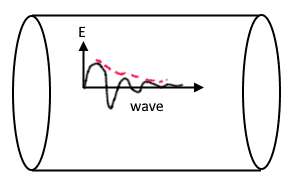Then, I realized if the wave direction is actually perpendicular to the length of the conductor (shown below), then it makes sense. So the E field is actually along the length of the conductor. Is this correct way of interpreting this?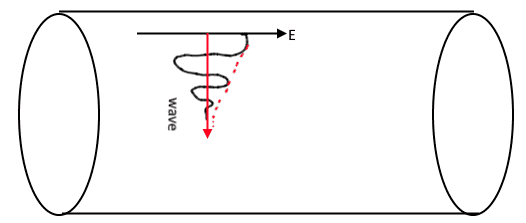Given that Electric field resides on the surface for a conductor, it makes perfect sense that current only travels on the surface, as the E field attenuates from the top.

#### Attachments

•Delta2

Homework Helper
Gold Member
Both cases make sense from a theoretical point of view.

It is just that if we have a cylindrical conductor that its length is long compared to its radius, it is more likely that it will be oriented in space in such a way that case in second scheme is the one that happens.

Last edited:
iVenky
Both cases make sense from a theoretical point of view.

It is just that if we have a cylindrical conductor that its length is long compared to its radius, it is more likely that it will be oriented in space in such a way that case in second scheme is the one that happens.

But wouldn't case 1 mean that E field can't propagate through the length of the cable at all after distance=skin depth? That means you can never have electric current through a wire, which doesn't make sense..What's that I am missing here?

Homework Helper
Gold Member
But wouldn't case 1 mean that E field can't propagate through the length of the cable at all after distance=skin depth? That means you can never have electric current through a wire, which doesn't make sense..What's that I am missing here?

I thought we were talking about the case of an external EM-wave as it meets the surface of a cylindrical conductor.

You are talking about the case where we have a voltage (or current source) that creates a current through the axis of the cylindrical conductor. This case is different. I think the voltage source forces some sort of longitudinal EM-wave along the axis of the cylindrical conductor. The skin depth now applies in direction perpendicular to the direction of this longitudinal EM wave, that is in the radial direction of the cylindrical conductor.

EDIT: Maybe my explanation is not satisfactory. I ll just mention @CWatters and @Charles Link and maybe they can explain better.

•iVenky
Gold Member
If electrons are moving back and forth longitudinally on the surface of the conductor, then they will radiate waves at right angles to the wire, both outward going and also into the wire.
Of course, the bulk of our energy flows along the wire, and is not radiated. There are two modes by which a wave travels along a wire: the TEM mode, where the electric field links to a neighbouring conductor, and the single-wire mode, where the electric field links to positions half a wavelength along the same wire.This mode is more common than is generally supposed, and results in a surface wave traveling along the surface of the wire, with another small wave propagating into the wire to satisfy the losses, as you have described.

iVenky
If electrons are moving back and forth longitudinally on the surface of the conductor, then they will radiate waves at right angles to the wire, both outward going and also into the wire.

is the one that results in E field along the length of the cable, right? What confusing me now if I assume flow of electrons through the inner core of the conductor (than outer surface), then we would still have E field inside, as it dissipates towards the border like shown below,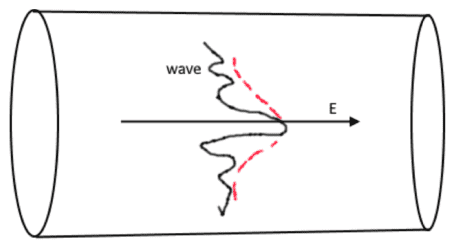What's the flaw in this logic.

Last edited:
Gold Member